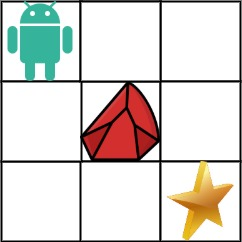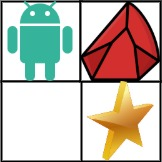[LeetCode][Medium] 63. Unique Paths II
2021-04-03
Engineering
2 MIN

## 題目

A robot is located at the top-left corner of a `m x n` grid (marked 'Start' in the diagram below).

The robot can only move either down or right at any point in time. The robot is trying to reach the bottom-right corner of the grid (marked 'Finish' in the diagram below).

Now consider if some obstacles are added to the grids. How many unique paths would there be?

An obstacle and space is marked as `1` and `0` respectively in the grid.

Example 1:``````Input: obstacleGrid = [[0,0,0],[0,1,0],[0,0,0]]
Output: 2
Explanation: There is one obstacle in the middle of the 3x3 grid above.
There are two ways to reach the bottom-right corner:
1. Right -> Right -> Down -> Down
2. Down -> Down -> Right -> Right
``````

Example 2:``````Input: obstacleGrid = [[0,1],[0,0]]
Output: 1
``````

## 思路

#62 一樣的方法，但要先檢查障礙物，遇到障礙物就變成0。

## Solution

``````/**
* @param {number[][]} obstacleGrid
* @return {number}
*/
var uniquePathsWithObstacles = function(obstacleGrid) {
var m = obstacleGrid.length;
var n = (obstacleGrid || []).length;
var dp = Array(m);
var left = 0;
var top = 0;

if (!m || !n) return 0;

for (var i = 0; i < m; i++) {
dp[i] = Array(n);
for (var j = 0; j < n; j++) {
left = (j === 0 || obstacleGrid[i][j - 1] === 1) ? 0 : dp[i][j - 1];
top = (i === 0 || obstacleGrid[i - 1][j] === 1) ? 0 : dp[i - 1][j];
dp[i][j] = obstacleGrid[i][j] === 1 ? 0 : ((i === 0 && j === 0) ? 1 : (left + top));
}
}

return dp[m - 1][n - 1];
};
``````

## Complexity

• Time complexity : O(m * n)
• Space complexity : O(m * n)